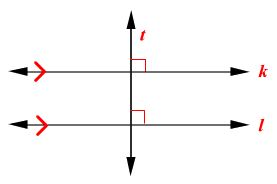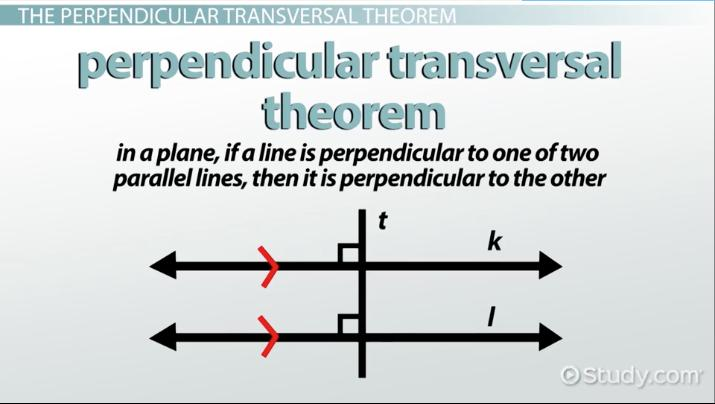# The Perpendicular Transversal Theorem & Its Converse

Lesson Transcript
Instructor: Yuanxin (Amy) Yang Alcocer

Amy has a master's degree in secondary education and has been teaching math for over 9 years. Amy has worked with students at all levels from those with special needs to those that are gifted.

The perpendicular transversal theorem states that if two parallel lines are in a plane, a line perpendicular to one line will be perpendicular to the other. Learn about this theorem, congruent angles, the converse of the theorem and parallel lines. Updated: 11/23/2021

## The Perpendicular Transversal Theorem

One of the interesting things we do in math is look at lines and angles, and we try to figure out a way to prove whether they are the same or have any other similarities. Even if two things look the same, mathematicians will never say they are unless they can prove it mathematically. That is where our theorems come in. A theorem is a proven statement. By using a theorem, mathematicians can prove a certain situation is definitely true or not. When mathematicians can prove that a certain situation is true, then they can move on and build on that information. This is how the field of mathematics has grown over the past hundreds of years. All the mathematics that we learn is based on layers and layers of mathematical proofs and theorems.

In this lesson, we'll look at the perpendicular transversal theorem and how you can use this theorem to help you find parallel lines. This particular theorem tells you that in a plane, if a line is perpendicular to one of two parallel lines, then it is perpendicular to the other. What does this mean? Take a look at these two lines.This theorem is telling you that line t is perpendicular to line k, and lines k and l are parallel, then line t is also perpendicular to line l.An error occurred trying to load this video.

Try refreshing the page, or contact customer support.

Coming up next: How to Find and Apply The Slope of a Line

### You're on a roll. Keep up the good work!

Replay
Your next lesson will play in 10 seconds
• 0:01 Perpendicular…
• 1:17 Two Congruent Angles
• 1:55 Converse of the Theorem
• 2:20 Two Parallel Lines
• 2:50 Lesson Summary
Save Save

Want to watch this again later?

Timeline
Autoplay
Autoplay
Speed Speed

## Two Congruent Angles

You can actually use this theorem to help you prove two angles are congruent, or two angles that are the same. How? Well, think about what it means to be perpendicular. When two lines are perpendicular, it means that they intersect at a right angle. So, the angles formed by the intersection of the two lines are right angles. If we have two intersections that are both perpendicular, then the corresponding angles are congruent to each other. Looking at our image, the two angles that are marked are congruent to each other.

To unlock this lesson you must be a Study.com Member.

### Register to view this lesson

Are you a student or a teacher?